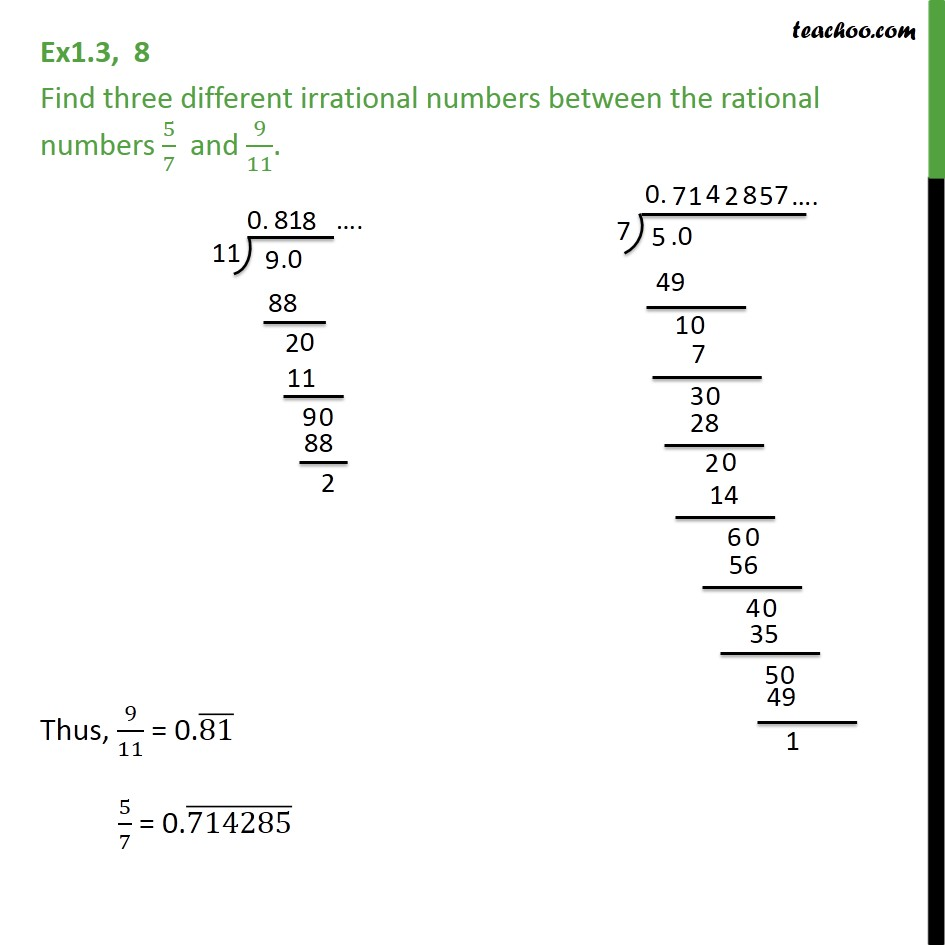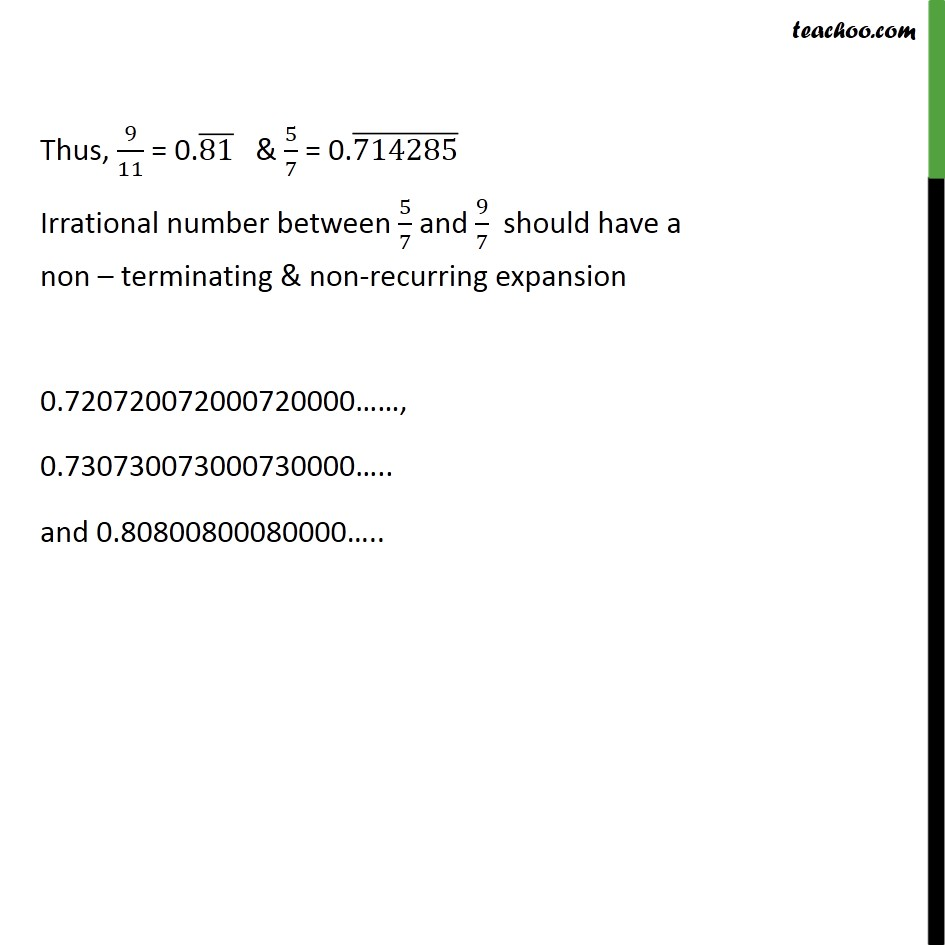1. Chapter 1 Class 9 Number Systems (Term 1)
2. Concept wise
3. Finding irrational numbers b/w two numbers

Transcript

Ex1.3, 8 Find three different irrational numbers between the rational numbers 5/7 and 9/11. Thus, 9/11 = 0.(81) 5/7 = 0.(714285) Thus, 9/11 = 0.(81) & 5/7 = 0.(714285) Irrational number between 5/7 and 9/7 should have a non terminating & non-recurring expansion 0.720720072000720000 , 0.730730073000730000 .. and 0.80800800080000 ..